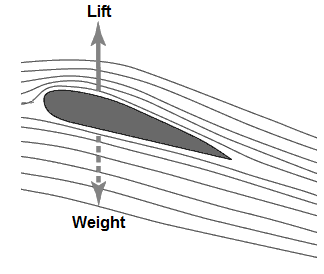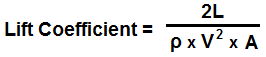﻿ Lift Coefficient Calculator ﻿# Lift Coefficient CalculatorLift Force, L: N Dynamic pressure, q Pa Area, A m2

nan

This lift coefficient calculator calculates the lift coefficient of an aerodynamic element based on the lift force, L, that the element is experiencing, the dynamic pressure, q, that the element is experiencing, and the area, A, that the element has exposed to generate aerodynamic lift.

The lift coefficient is a dimensionless unit that relates the lift force to the dynamic pressure and the area. Based on this ratio, the lift coefficient is taken.

The lift force, L, is the amount of force that is applied upward to an aerodynamic element so that it can overcome its element of weight. For an airplane to go airborne, the force of lift must be greater than the force of weight. The greater the lift force, the greater the coefficient of lift of an aerodynamic element.

The dynamic pressure, q, is the force that is created by an aerodynamic element in motion. Dynamic pressure is equal to one-half times the velocity squared (q= 0.5ρV2). Dynamic pressure is created when the aerodynamic element is in motion. Thus, the greater the velocity of the element, meaning the greater speed at which it is travelling, the greater the dynamic pressure. This is why an airplane is able to generate more lift while it is travelling fast and must maintain a certain airspeed in order to be in flight. Otherwise, sufficient lift will not be generated and the airplane will stall in flight and fall out of the air. Since the lift coefficient is referenced from the point of view of the lift force, the greater the dynamic pressure, the smaller the lift coefficient.

The area, A, is the amount of surface area that can create lift that the aerodynamic element exposes. The greater the area, the more lift that can be created. This is why airplanes have very large wings. The wings have large surface area. This gives a lot of area for an upward force to act on the wings to lift the aircraft. The larger the surface area, the more potential for more lift. Since, again, the lift coefficient is referenced in regard to the lift force, the larger the area, the smaller the lift coefficient.

To use this calculator, a user just enters the lift force, L, of the aerodynamic element, its dynamic pressure, q, and the area of its wings, and presses the 'Calculate' button. The resultant value will then be automatically computed and shown below. The resultant lift coefficient again has no units.

The lift coefficient is a number that aviation engineers use to model the aerodynamic ability of an aerodynamic element. The greater the coeffiicent, the more aerodynamic an element is in regards to generating lift.

### Lift Coefficient Calculator if Dynamic Pressure is Not KnownLift Force, L: N Density, ρ kg/m3 Velocity, V m/s Area, A m2

nan

This calculator also calculates the lift coefficient if the dynamic pressure is not known upright.

Since dynamic pressure is equal to one-half density times velocity squared, it is substituted into the original formula. This gives us q= 2L/ρV2A.

To use this calculator, a user just enters the lift force, L, the density, ρ, of the fluid the aerodynamic element is moving through, the velocity, v, at which the aerodynamic element is travelling through the fluid, and the area, A, of the aerodyamic elements lift structure. Once the 'Calculate' button is pressed, the resultant value will be computed and displayed.

Related Resources

﻿